Question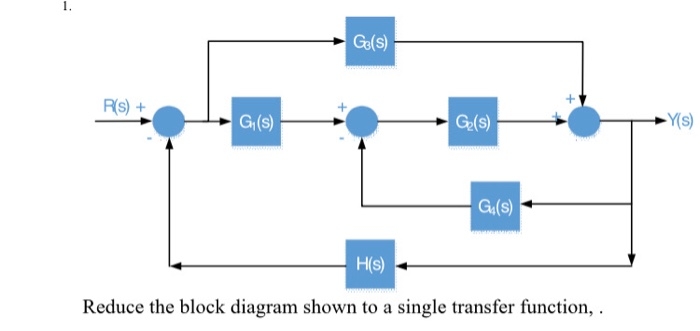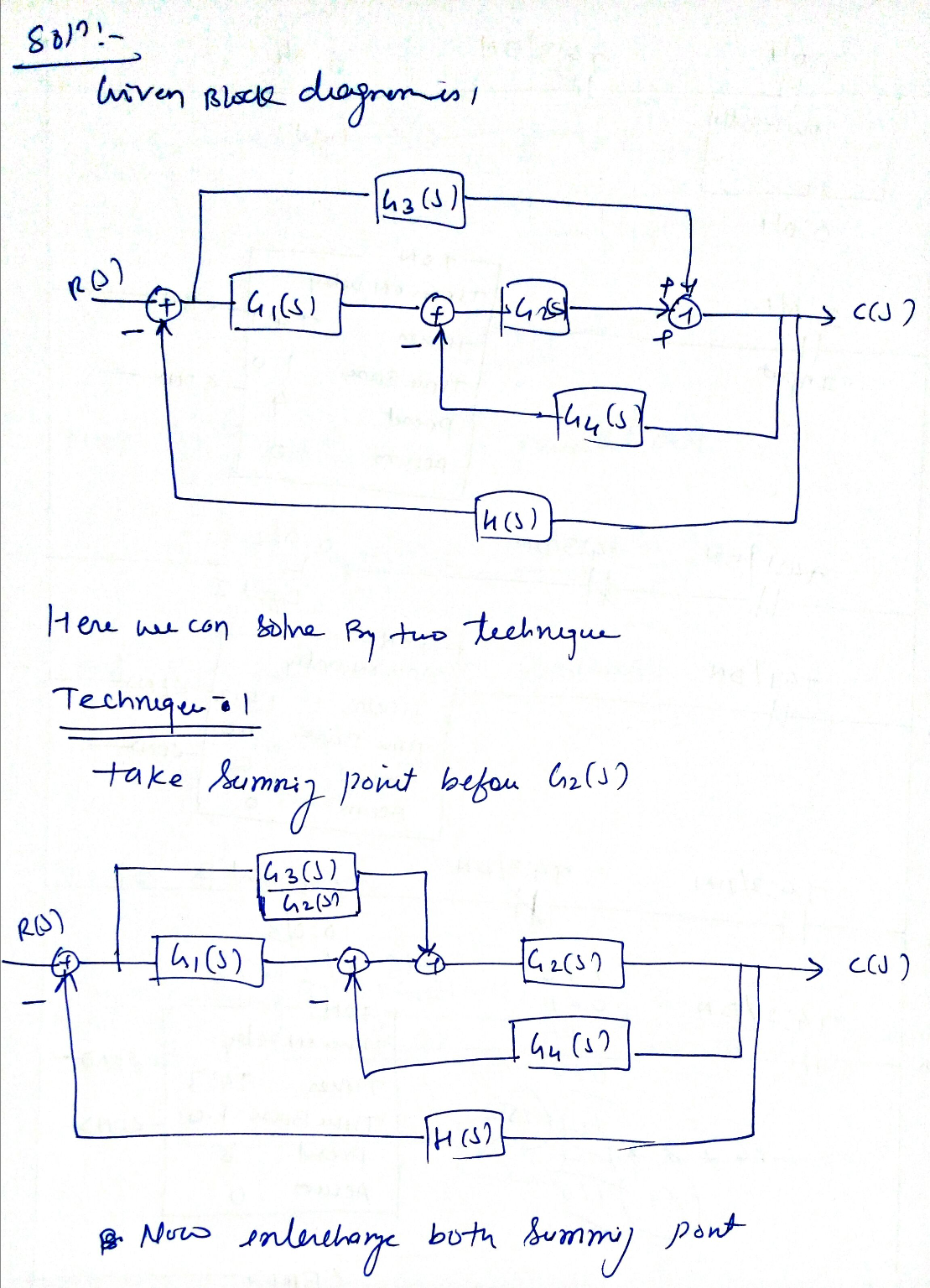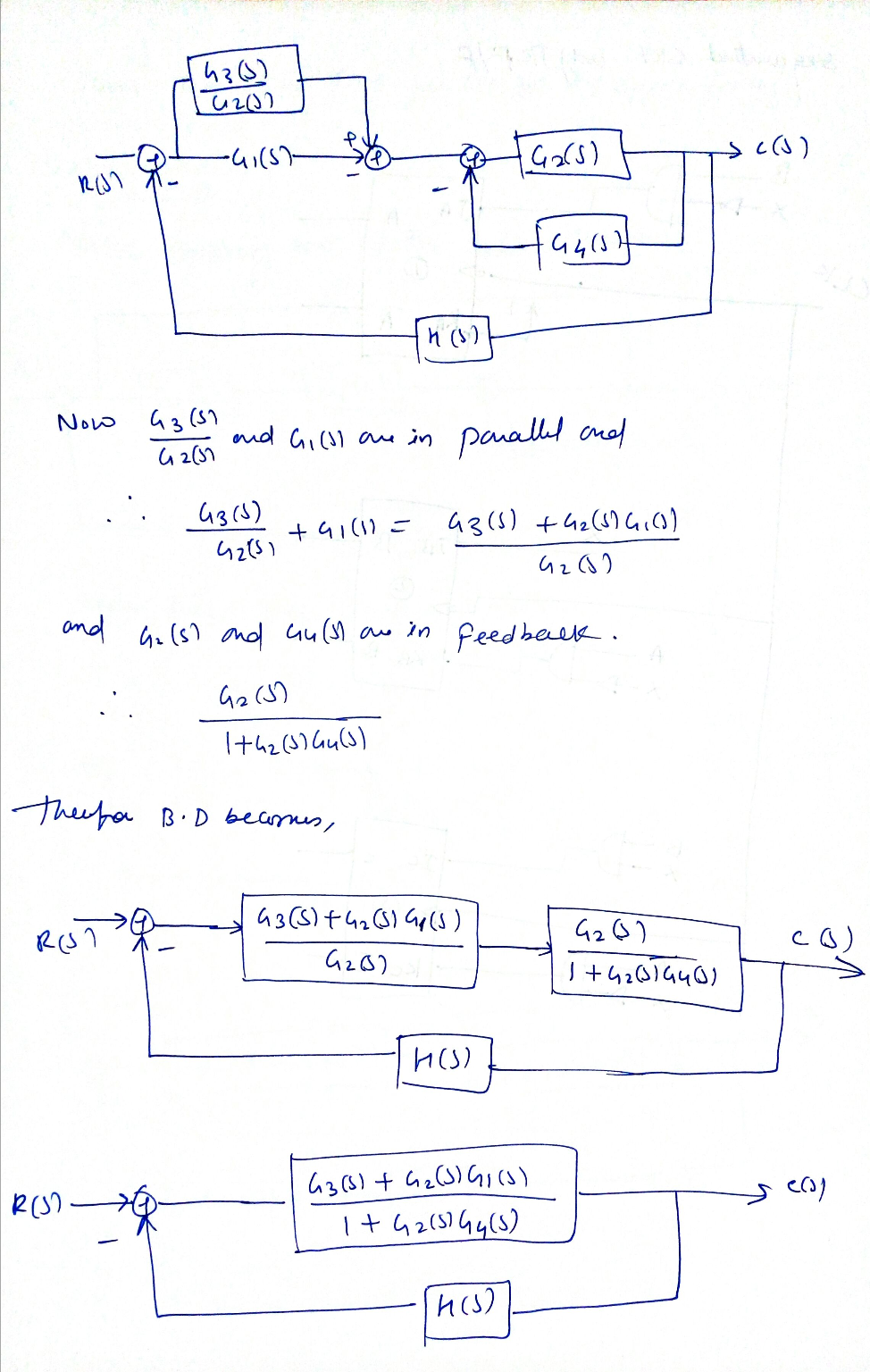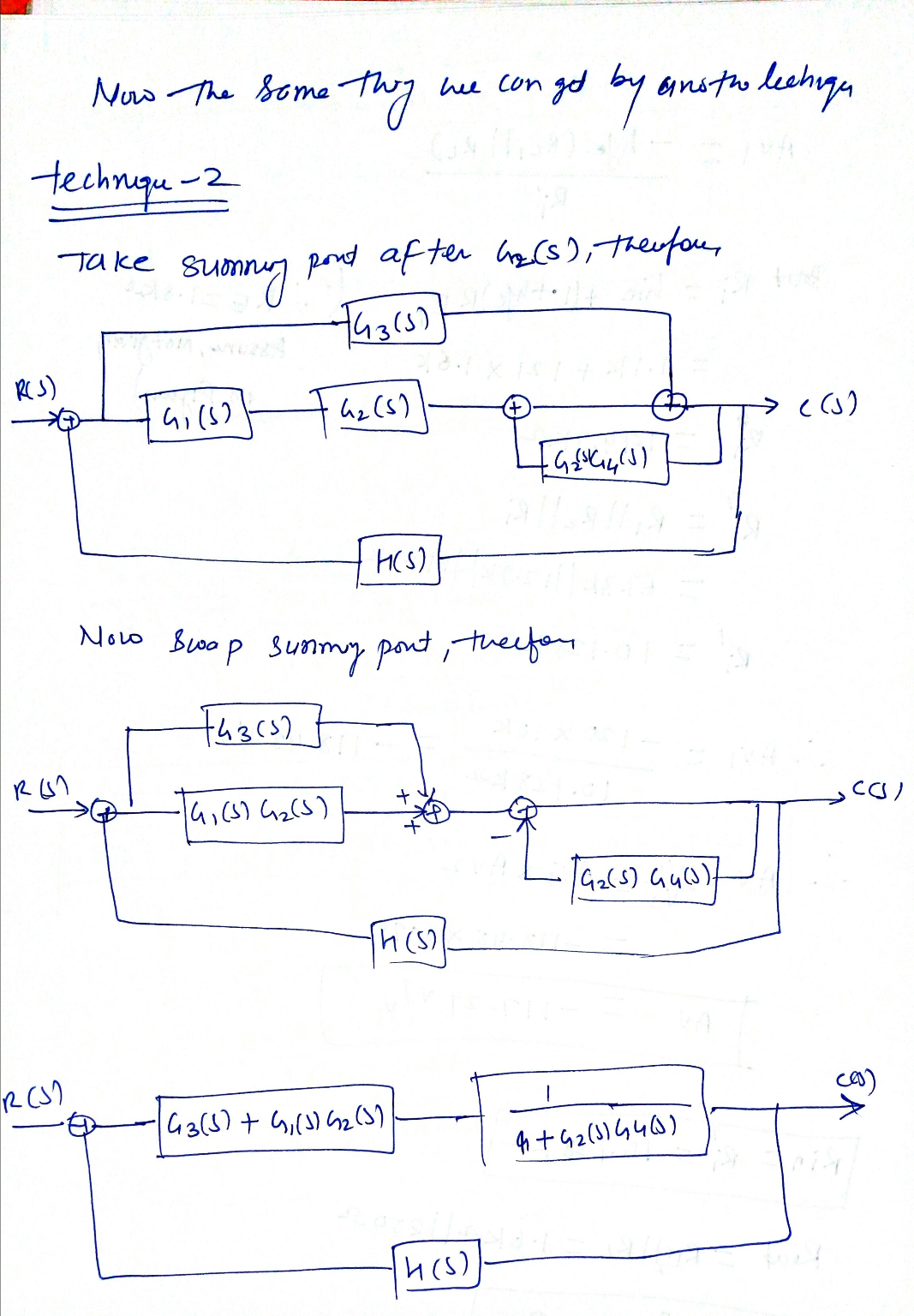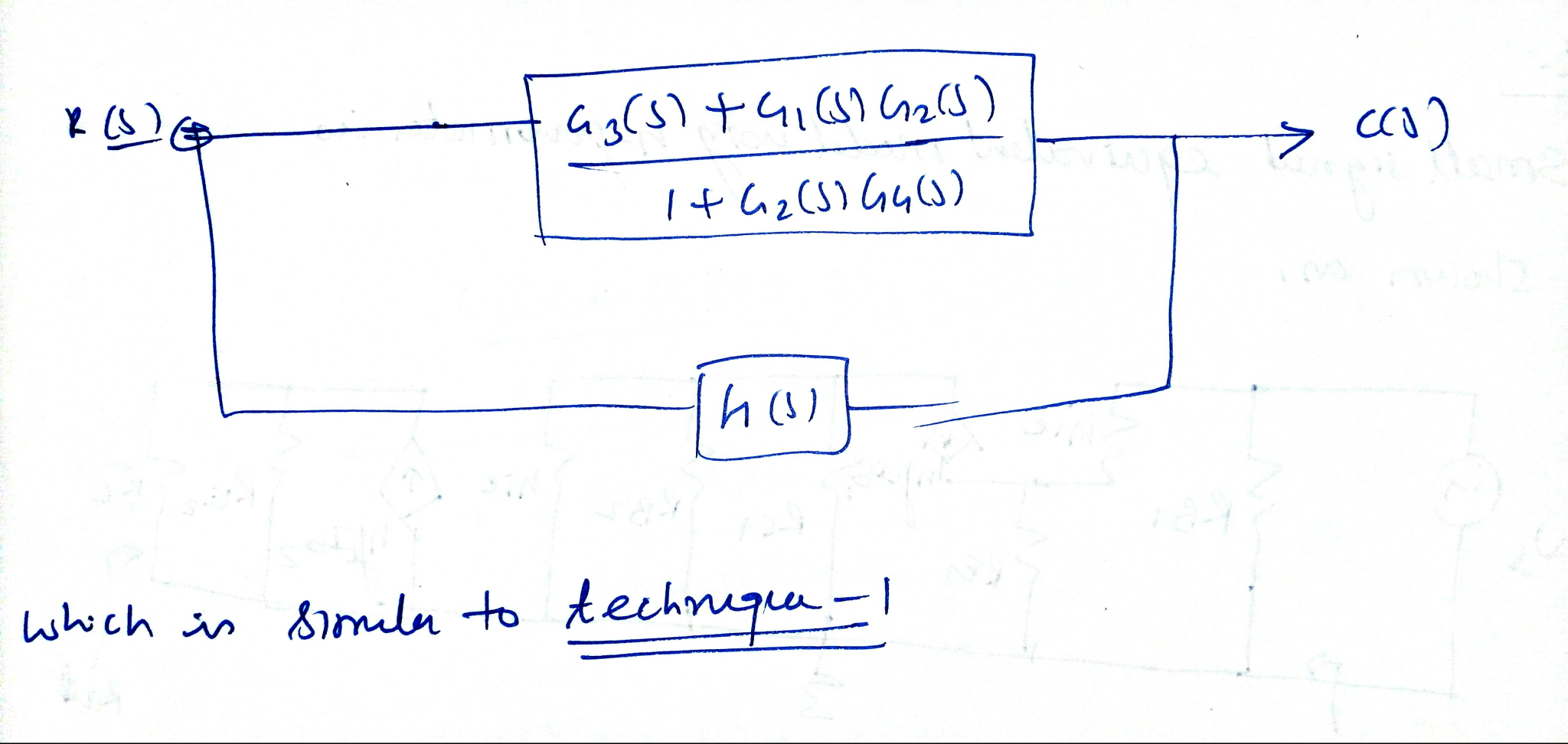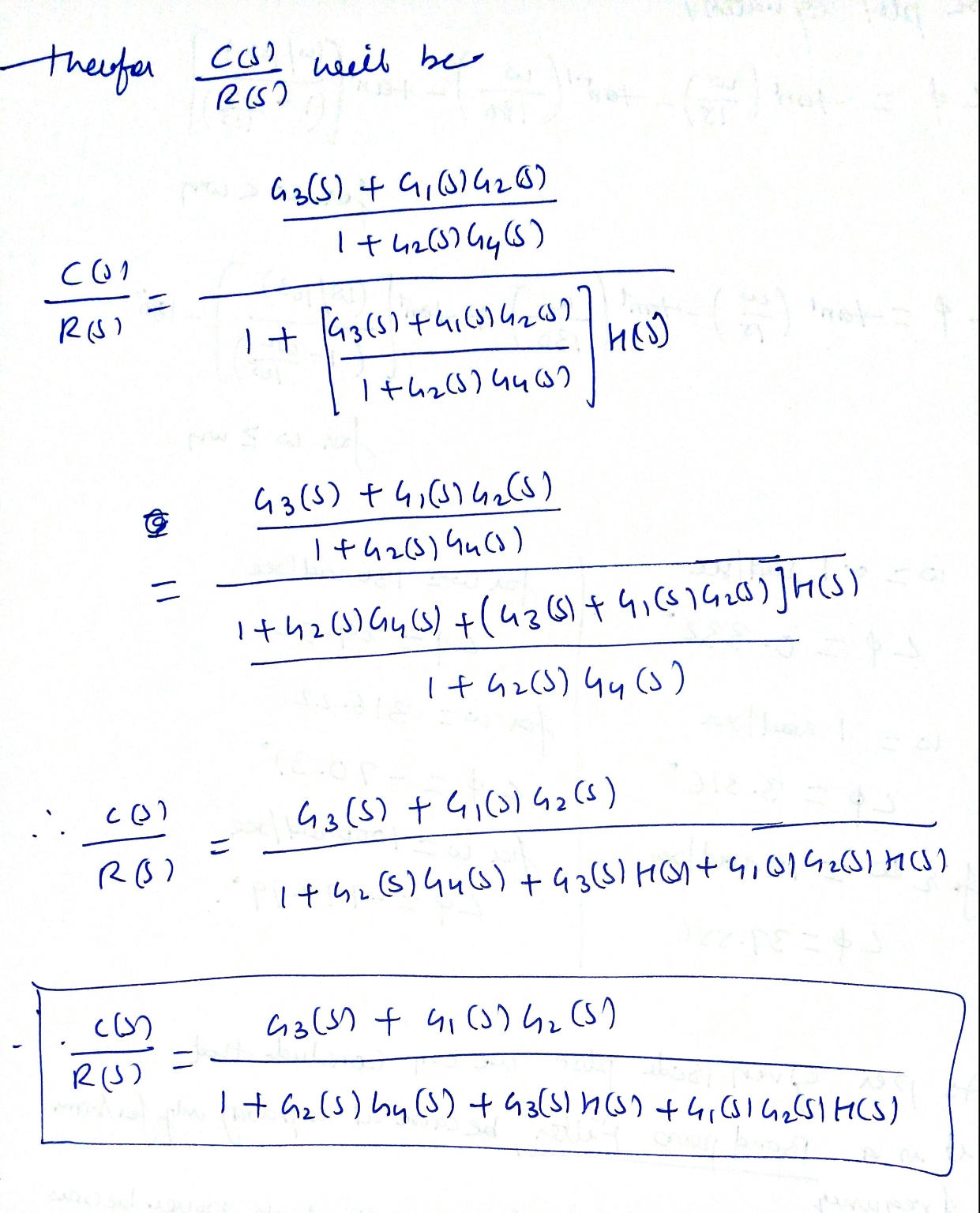Note-please find me in comment box if you find any difficulty. I have given you two techniques for reducing block diagram, so you can learn better. You find it helpful please thumbs up. Good luck

#### Earn Coins

Coins can be redeemed for fabulous gifts.

Similar Homework Help Questions
• ### Reduce the block diagram shown to a single transfer function, T(s) C(s)/R(s) Gl (s) G2(s) G3(s)...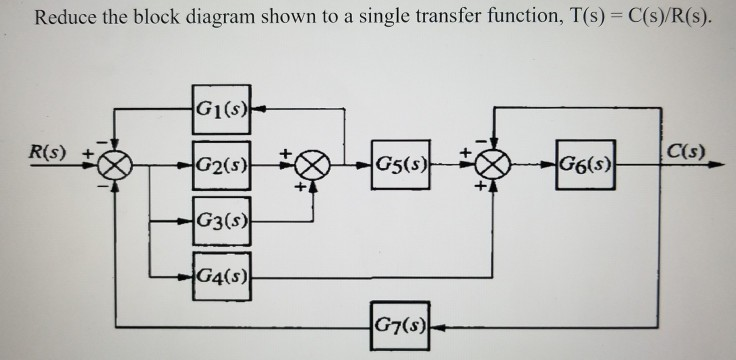Reduce the block diagram shown to a single transfer function, T(s) C(s)/R(s) Gl (s) G2(s) G3(s) G4(s) C(s) G6(s) G7(s)

• ### Reduce the block diagram below to a single block representing the transfer function T(S) C(s) R(S)...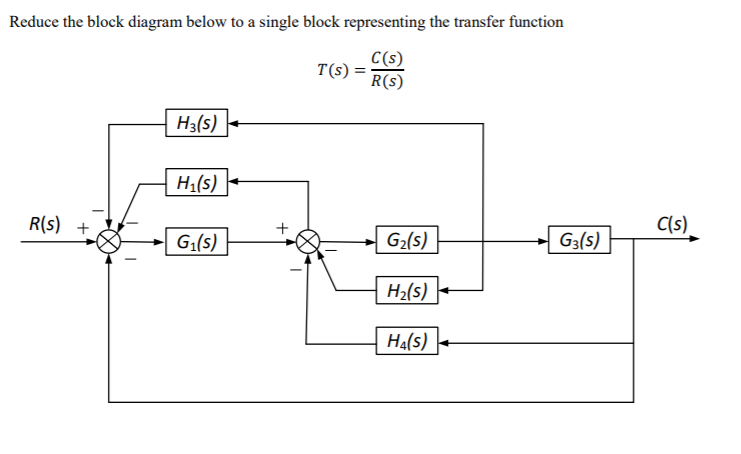Reduce the block diagram below to a single block representing the transfer function T(S) C(s) R(S) H3(s) Hl(s) R(s) + C(s) Gi(s) G2(s) G3(s) Hz(s) H4(s)

• ### Question #2 ( 25 points) C(s) a) Reduce the block diagram shown in Figure 1 to a single transfer function T(s) =R)...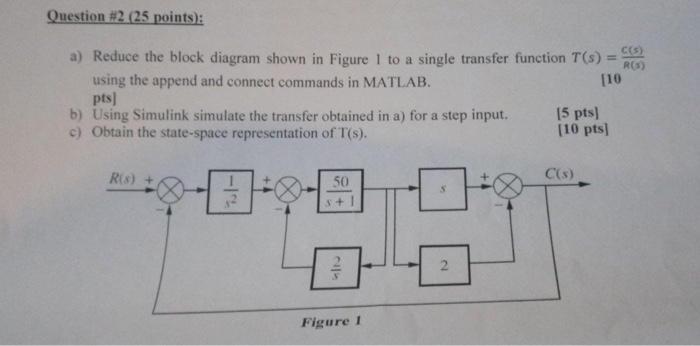Question #2 ( 25 points) C(s) a) Reduce the block diagram shown in Figure 1 to a single transfer function T(s) =R) using the append and connect commands in MATLAB. pts b) Using Simulink simulate the transfer obtained in a) for a step input. c) Obtain the state-space representation of T(s). [10 [5 pts [10 pts] C(s) Ris 50 s+I 2 Figure 1 -Irt Question #2 ( 25 points) C(s) a) Reduce the block diagram shown in Figure 1 to...

• ### Reduce the block diagram shown to a single block T(s)= C(s)/R(s). TICCll.. A ram shown in...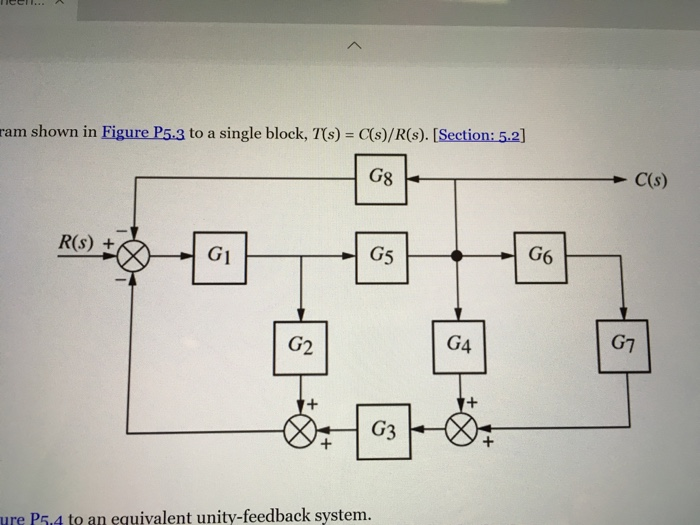Reduce the block diagram shown to a single block T(s)= C(s)/R(s). TICCll.. A ram shown in Figure P5.3 to a single block, T/s) = C(s)/R(s). [Section: 5.2] G8 C(s) R(S) + G6 G3 ure P5.4 to an equivalent unity-feedback system.

• ### R E UA Given the block diagram as shown, R and D are inputs and G,...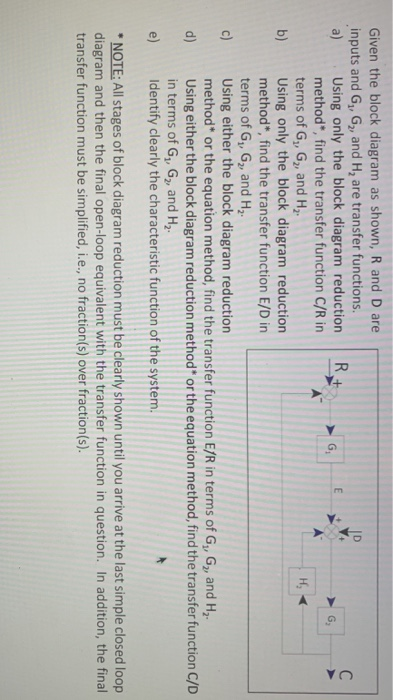R E UA Given the block diagram as shown, R and D are inputs and G, G, and H, are transfer functions. a) Using only the block diagram reduction G G method", find the transfer function C/R in terms of G, G, and H. H, b) Using only the block diagram reduction method*, find the transfer function E/D in terms of G, G, and Hz. c) Using either the block diagram reduction method* or the equation method, find the transfer...

• ### 03. (a) Consider the block diagram shown in Figure 3.1, and assume G(s)= 3. G,(s) and...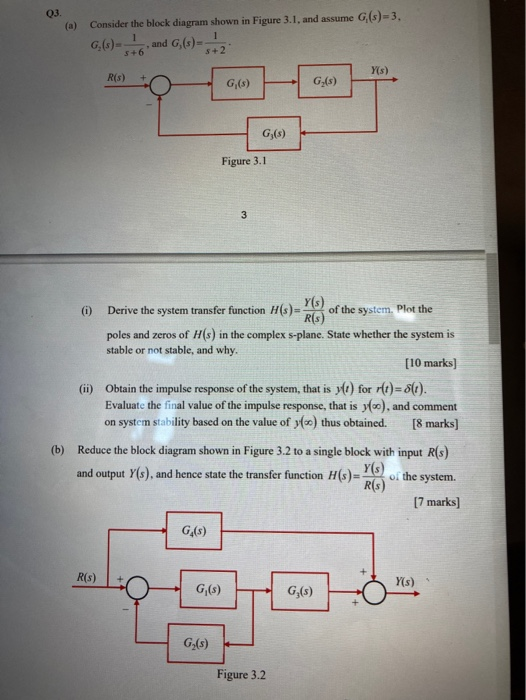03. (a) Consider the block diagram shown in Figure 3.1, and assume G(s)= 3. G,(s) and G,(s) 5+2 Y(s) R(S) G,() Gy(s) G;(s) Figure 3.1 3 (0) Y(s) Derive the system transfer function H(s)= of the system. Plot the R(s) poles and zeros of H(s) in the complex s-plane. State whether the system is stable or not stable, and why. [10 marks) (11) Obtain the impulse response of the system, that is ylt) for r(t)= 8(t). Evaluate the final value...

• ### [5.0 Marks] Simplify the block-diagram shown in Figure 1 and reduce it to a single simplified...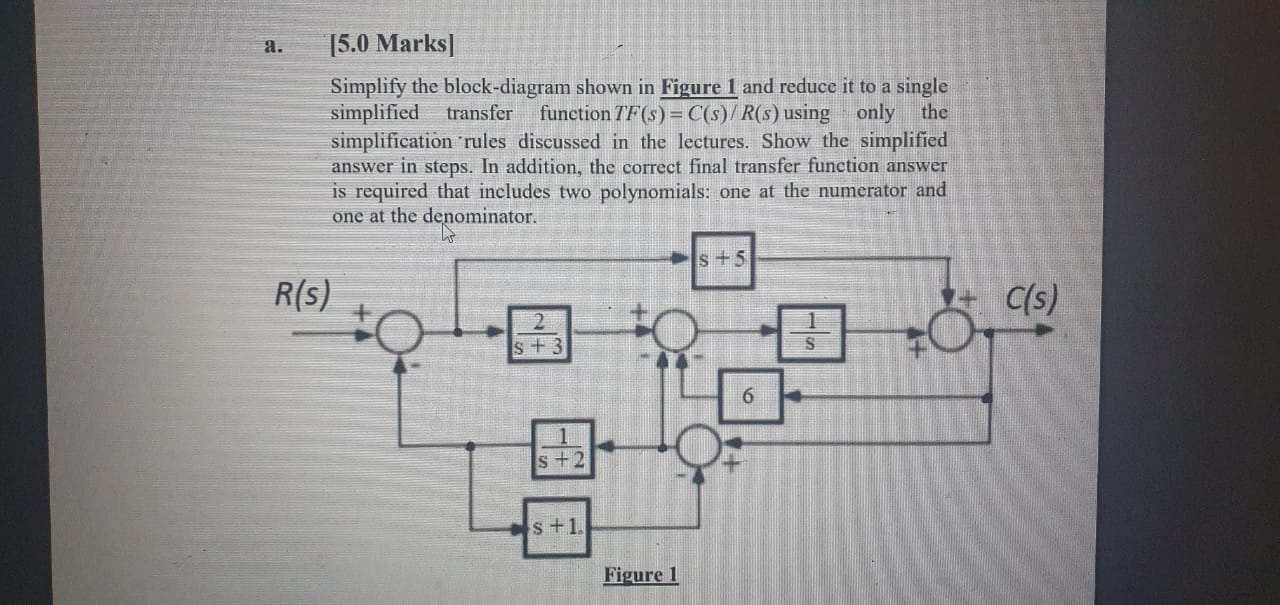[5.0 Marks] Simplify the block-diagram shown in Figure 1 and reduce it to a single simplified transfer function TF(s) = C(s)/R(s) using only the simplification 'rules discussed in the lectures. Show the simplified answer in steps. In addition, the correct final transfer function answer is required that includes two polynomials: one at the numerator and one at the denominator. s + 5 R(s) - C(s) L. s + 3 S 6 s + 2 s +1. Figure 1

• ### C(s) R(s) 5 Q2: Reduce the block diagram and find the transfer function: C(s) 3 4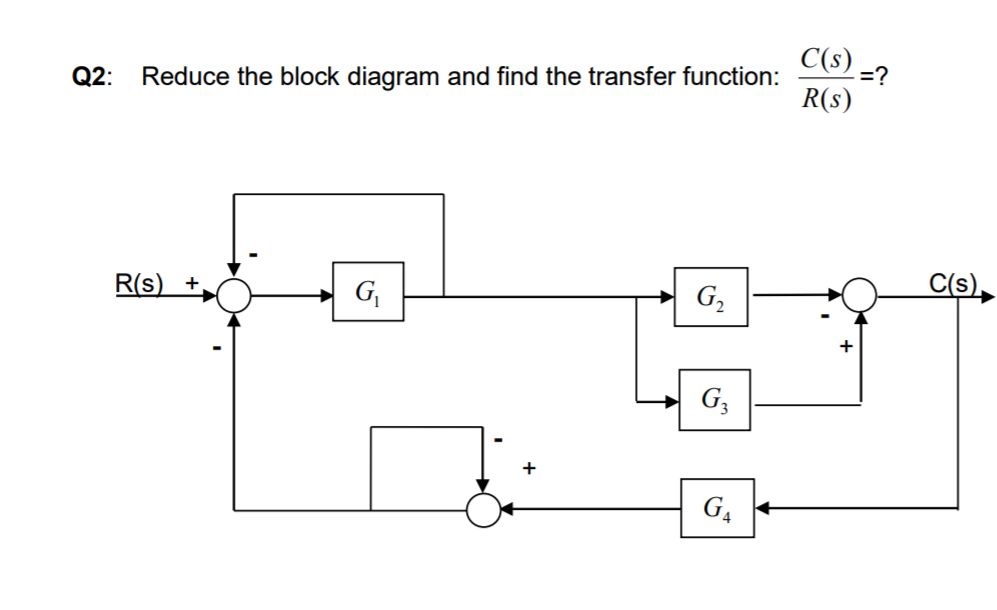C(s) R(s) 5 Q2: Reduce the block diagram and find the transfer function: C(s) 3 4

• ### Section VI: Block Diagram Reduction Individually reduce each of the following Block Diagrams into a single...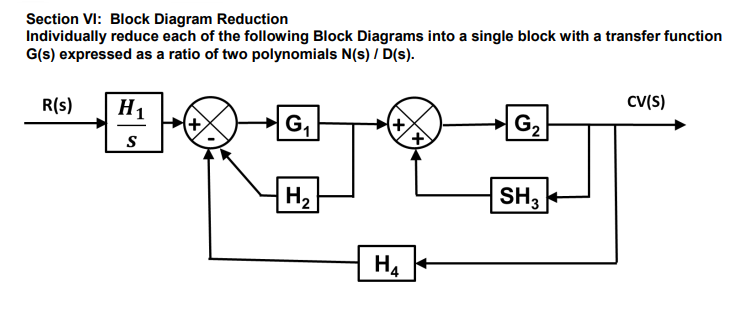Section VI: Block Diagram Reduction Individually reduce each of the following Block Diagrams into a single block with a transfer function G(s) expressed as a ratio of two polynomials N(s) / D(s) CV(S) R(s) H1 SH3 2 4

• ### Q4. (a) Reduce the block diagram shown in Figure Q4a to a single mathematical expression suitable for implementation in MATLAB. Each letter represents a transfer function in the s-domain. (...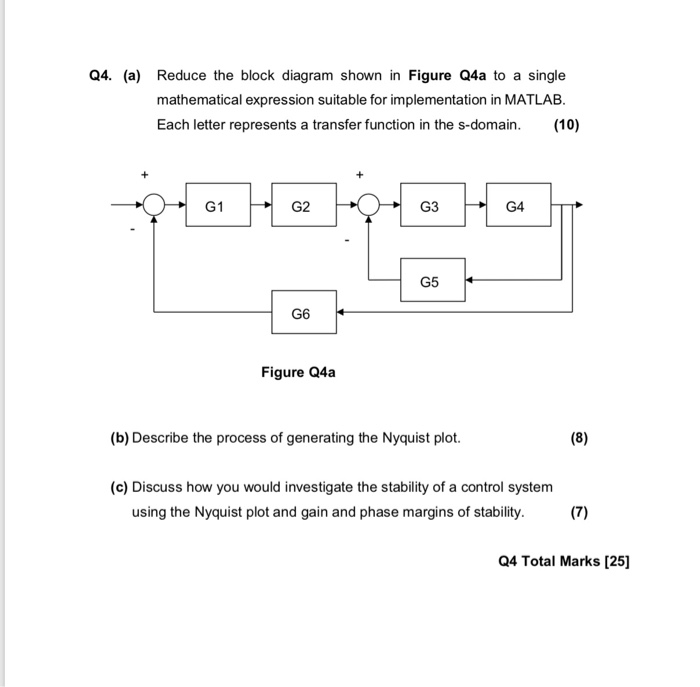Q4. (a) Reduce the block diagram shown in Figure Q4a to a single mathematical expression suitable for implementation in MATLAB. Each letter represents a transfer function in the s-domain. (10) G1 G2 G3 G4 G5 G6 Figure Q4a (b) Describe the process of generating the Nyquist plot. (c) Discuss how you would investigate the stability of a control system using the Nyquist plot and gain and phase margins of stability. (7) Q4 Total Marks  educe the block diagram shown...